# A reducing elbow is used to deflect water flow at a rate of 30 kg/s in a horizontal pipe upward by an angle θ = 45° from the flow direction while accelerating it. The elbow discharges water into the atmosphere. The cross- sectional area of the elbow is 150 cm2 at the inlet and 25 cm2 at the exit. The elevation difference between the centers of the exit and the inlet is 40 cm. The mass of the elbow and the water in it is 50 kg. Determine the anchoring force needed to hold the elbow in place. Take the momentum-flux correction factor to be 1.03.

Question-AnswerCategory: Fluid MechanicsA reducing elbow is used to deflect water flow at a rate of 30 kg/s in a horizontal pipe upward by an angle θ = 45° from the flow direction while accelerating it. The elbow discharges water into the atmosphere. The cross- sectional area of the elbow is 150 cm2 at the inlet and 25 cm2 at the exit. The elevation difference between the centers of the exit and the inlet is 40 cm. The mass of the elbow and the water in it is 50 kg. Determine the anchoring force needed to hold the elbow in place. Take the momentum-flux correction factor to be 1.03.

A reducing elbow is used to deflect water flow at a rate of 30 kg/s in a horizontal pipe upward by an angle θ = 45° from the flow direction while accelerating
it. The elbow discharges water into the atmosphere. The cross- sectional area of the elbow is 150 cm2 at the inlet and 25 cm2 at the exit. The elevation difference between the centers of the exit and the inlet is 40 cm. The mass of the elbow and the water in it is 50 kg. Determine the anchoring force needed to hold the elbow in place. Take the momentum-flux correction factor to be 1.03.Step: 1

Given data:-
The cross sectional area at inlet is.
The cross sectional area at exit is.
The elevation difference is.
The angle is.
The mass of elbow and the water in it is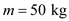.
The momentum-flux correction factor for each inlet and outlet is.

Step: 2

Assumptions
1. The flow is steady, frictionless, incompressible, and irrotational.
2. Water is discharged to the atmosphere, and thus the gage pressure at the outlet is zero. 3. Let the mass flow rate beProperties: Take the density of water as 1000 kg/m3.

Step: 3

Take the elbow as the control volume. Now, the inlet is at point 1 and the outlet at point 2.
Now,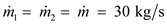,
Since the flow is steady. Also,.
Now, the inlet velocity is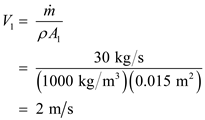Now, the outlet velocity isStep: 4

Now, use Bernoulli’s equation,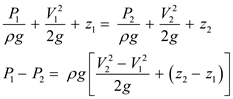Thus,Step: 5

Let the anchoring force of the elbow in x direction be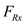and anchoring force of the elbow in z direction be. Now, the momentum equation for steady one-dimensional flow,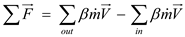.
Now, the components of the equation in x direction is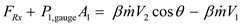Step: 6

Now, the components of the equation in z direction isNow, weight of the elbow and the water in it is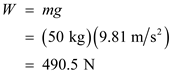Now, substitute the known values and solve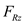.Now,
The resultant forceStep: 7

Now, the angle,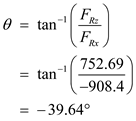Therefore, the anchoring force is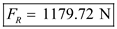. The force is acting at an angle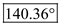from the positive x direction.